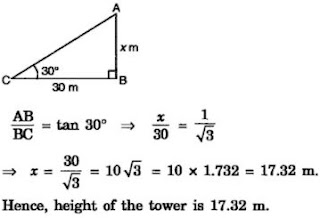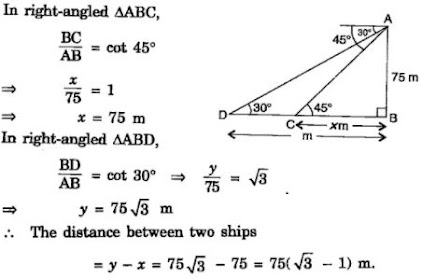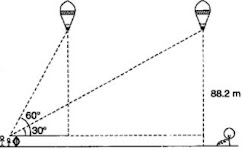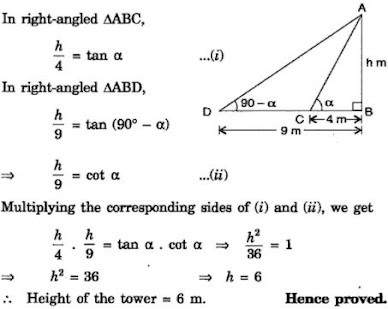# NCERT Solution for Class 10 Mathematics Chapter 9 Some Applications of Trigonometry

 Chapter Name NCERT Solution for Class 10 Maths Chapter 9 Some Applications of Trigonometry Topics Covered Short Revision for the ChapterNCERT Exercise Solution Related Study NCERT Solution for Class 10 MathsNCERT Revision Notes for Class 10 MathsImportant Questions for Class 10 MathsMCQ for Class 10 MathsNCERT Exemplar Questions For Class 10 Maths

## Short Revision for Some Applications of Trigonometry

1. The height of an object and the distance between two distinct objects can be determined by using the trigonometric ratios.
2. The line of sight is the line drawn from the eye of an observer to the point in the object.
3. The angle of elevation is the angle formed by the line of sight with the horizontal when the object is above the horizontal level.
4. The angle of depression is the angle formed by the line of sight with the horizontal when the object is below the horizontal level.

### NCERT Exercise Solutions

#### Exercise 9.1

1. A circus artist is climbing a 20 m long rope, which is tightly stretched and tied from the top of a vertical pole to the ground. Find the height of the pole, if the angle made by the rope with the ground level is 30°. (see fig. 9.11)

Solution
Let height of the pole AB  = x m
Length of the rope AC = 20 m
In △ABC, ∠ACB = 30°
∴ sin 30° = AB/AC ⇒ 1/2 = x/20 ⇒ x = 10 m
∴ Height of the pole = 10 m.

2. A tree breaks due to storm and the broken part bends so that the top of the tree touches the ground making an angle 30° with it. The distance between the foot of the tree to the point where the top touches the ground is 8 m. Find the height of the tree.

Solution

Let the height of tree before storm be AB. Due to storm it breaks from C such that its top A touches the ground at D and makes an angle of 30° .3. A contractor plans to install two slides for the children to play in a park. For the children below the age of 5 years, she prefers to have a slide whose top is at a height of 1.5 m, and is inclined at an angle of 30° to the ground, whereas for elder children, she wants to have a steep slide at a height of 3m, and inclined at an angle of 60° to the ground. What should be the length of the slide in each case?

4. The angle of elevation of the top of a tower from a point on the ground, which is 30 m away from the foot of the tower, is 30°. Find the height of the tower.

Solution

Let AB be the tower and C be a point on the ground such that BC = 30 m. Also let AB = x m.
The angle of elevation of the top A from C is 30° .
So, in right - angled △ABC,5. A kite is flying at a height of 60 m above the ground. The string attached to the kite is temporarily tied to a point on the ground. The inclination of the string with the ground is 60°. Find the length of the string, assuming that there is no slack in the string.

6. A 1.5 m tall boy is standing at some distance from a 30 m tall building. The angle of elevation from his eyes to the top of the building increases from 30° to 60° as he walks towards the building. Find the distance he walked towards the building.

Solution

Let a 1.5 m tall boy DP finds the angle of elevation to the top A of the building A.
Let DCB be the horizontal eye sight. When that boy walks towards the building finds increased angle of 60° at C.7. From a point on the ground, the angles of elevation of the bottom and the top of a
transmission tower fixed at the top of a 20 m high building are 45° and 60° respectively. Find the height of the tower.

8. A statue, 1.6 m tall, stands on the top of a pedestal. From a point on the ground, the angle of elevation of the top of the statue is 60° and from the same point the angle of elevation of the top of the pedestal is 45°. Find the height of the pedestal.

9. The angle of elevation of the top of a building from the foot of the tower is 30° and the angle of elevation of the top of the tower from the foot of the building is 60°. If the tower is 50 m high, find the height of the building.

10. Two poles of equal heights are standing opposite each other on either side of the road, which is 80 m wide. From a point between them on the road, the angles of elevation of the top of the poles are 60° and 30°, respectively. Find the height of the poles and the distances of the point from the poles.

11. A TV tower stands vertically on a bank of a canal. From a point on the other bank directly opposite the tower, the angle of elevation of the top of the tower is 60°. From another point 20 m away from this point on the line joing this point to the foot of the tower, the angle of elevation of the top of the tower is 30° (see Fig. 9.12). Find the height of the tower and the width of the canal.

12. From the top of a 7 m high building, the angle of elevation of the top of a cable tower is 60° and the angle of depression of its foot is 45°. Determine the height of the tower.

Solution

In the figure, AB represents a 7 m high building and PQ represents a cable tower. Angle of elevation to the top P from A is 60° and angle of depression to the bottom Q from same point A is 45°.

13. As observed from the top of a 75 m high lighthouse from the sea-level, the angles of depression of two ships are 30° and 45°. If one ship is exactly behind the other on the same side of the lighthouse, find the distance between the two ships.

Solution

In the figure, AB represents a 75 m high lighthouse, C and D are the positions of two ships where angles of depression from top of the tower are 45° and 30° respectively.
Let BC = x m and BD = y m.
We have to find the distance between the to ships,
i.e., CD = BD - BC = y - x14. A 1.2 m tall girl spots a balloon moving with the wind in a horizontal line at a height of 88.2 m from the ground. The angle of elevation of the balloon from the eyes of the girl at any instant is 60°. After some time, the angle of elevation reduces to 30° (see Fig. 9.13). Find the distance travelled by the balloon during the interval.Solution

When the horizontally moving balloon is at P, a girl AB (say) finds ∠PAC is 60° and at the position R, ∠RAD is 30° .
We have to find the distance travelled in horizontal line, i.e., PR of CD.
Consider PC = PQ - CQ = (88.2 - 1.2)m = 87 m = RD15. A straight highway leads to the foot of a tower. A man standing at the top of the tower observes a car at an angle of depression of 30°, which is approaching the foot of the tower with a uniform speed. Six seconds later, the angle of depression of the car is found to be 60°. Find the time taken by the car to reach the foot of the tower from this point.

Solution

Let AB be the tower on the top of which a man is standing and finds angle of depression to the position D of the running car as 30° .
After 6 seconds, angle is found 60° at C.
Let AB = h m, DC = x m and BC = y m.16. The angles of elevation of the top of a tower from two points at a distance of 4 m and 9 m from the base of the tower and in the same straight line with it are complementary. Prove that the height of the tower is 6 m.

Solution

In the drawn figure, AB represents a tower, C and D are two points distant 4 m and 9 m away from the base B.
Let angle of elevation to the top A from C is α and from D is 90° - α .